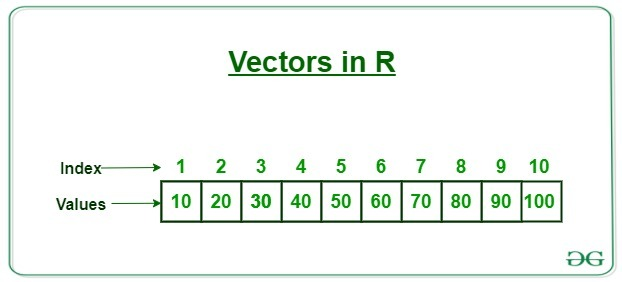# R – Vector

• Last Updated : 22 Apr, 2020

R programming is one of the most popular languages when it comes to data science, statistical computations or scientific research. R programming is widely used in machine learning and it is very efficient and user-friendly. It provides flexibility in doing big statistical operations with a few lines of code.

Vectors in R are the same as the arrays in C language which are used to hold multiple data values of the same type. One major key point is that in R the indexing of the vector will start from ‘1’ and not from ‘0’. We can create numeric vectors and character vectors as well.#### Types of vectors

Vectors are of different types which are used in R. Following are some of the types of vectors:

• Numeric vectors
Numeric vectors are those which contain numeric values such as integer, float, etc.

 `# R program to create numeric Vectors`` ` `# creation of vectors using c() function.``v1 <``-` `c(``4``, ``5``, ``6``, ``7``)`` ` `# display type of vector``typeof(v1)`` ` `# by using 'L' we can specify that we want integer values.``v2 <``-` `c(``1L``, ``4L``, ``2L``, ``5L``) `` ` `# display type of vector``typeof(v2)`

Output:

``` "double"
 "integer"```
• Character vectors
Character vectors contain alphanumeric values and special characters.
 `# R program to create Character Vectors`` ` `# by default numeric values ``# are converted into characters``v1 <``-` `c(``'geeks'``, ``'2'``, ``'hello'``, ``57``) `` ` `# Displaying type of vector``typeof(v1)`

Output:

` "character"`
• Logical vectors
Logical vectors contain boolean values such as TRUE, FALSE and NA for Null values.
 `# R program to create Logical Vectors`` ` `# Creating logical vector``# using c() function``v1 <``-` `c(TRUE, FALSE, TRUE, NA)`` ` `# Displaying type of vector``typeof(v1)`

Output:

` "logical"`

#### Creating a vector

There are different ways of creating vectors. Generally, we use ‘c’ to combine different elements together.

 `# R program to create Vectors`` ` `# we can use the c function``# to combine the values as a vector.``# By default the type will be double``X <``-` `c(``61``, ``4``, ``21``, ``67``, ``89``, ``2``)``cat(``'using c function'``, X, ``'\n'``)`` ` `# seq() function for creating``# a sequence of continuous values.``# length.out defines the length of vector.``Y <``-` `seq(``1``, ``10``, length.out ``=` `5``) ``cat(``'using seq() function'``, Y, ``'\n'``) `` ` `# use':' to create a vector ``# of continuous values.``Z <``-` `2``:``7``cat(``'using colon'``, Z)`

Output:

```using c function 61 4 21 67 89 2
using seq() function 1 3.25 5.5 7.75 10
using colon 2 3 4 5 6 7
```

#### Accessing vector elements

Accessing elements in a vector is the process of performing operation on an individual element of a vector. There are many ways through which we can access the elements of the vector. The most common is using the ‘[]’, symbol.

Note: Vectors in R are 1 based indexing unlike the normal C, python, etc format.

 `# R program to access elements of a Vector`` ` `# accessing elements with an index number.``X <``-` `c(``2``, ``5``, ``18``, ``1``, ``12``)``cat(``'Using Subscript operator'``, X[``2``], ``'\n'``)`` ` `# by passing a range of values``# inside the vector index.``Y <``-` `c(``4``, ``8``, ``2``, ``1``, ``17``)``cat(``'Using combine() function'``, Y[c(``4``, ``1``)], ``'\n'``)`` ` `# using logical expressions``Z <``-` `c(``5``, ``2``, ``1``, ``4``, ``4``, ``3``)``cat(``'Using Logical indexing'``, Z[Z>``4``])`

Output

```Using Subscript operator 5
Using combine() function 1 4
Using Logical indexing 5
```

#### Modifying a vector

Modification of a Vector is the process of applying some operation on an individual element of a vector to change its value in the vector. There are different ways through which we can modify a vector:

 `# R program to modify elements of a Vector`` ` `# Creating a vector``X <``-` `c(``2``, ``7``, ``9``, ``7``, ``8``, ``2``)`` ` `# modify a specific element``X[``3``] <``-` `1``X[``2``] <``-``9``cat(``'subscript operator'``, X, ``'\n'``)`` ` `# Modify using different logics.``X[X>``5``] <``-` `0``cat(``'Logical indexing'``, X, ``'\n'``)`` ` `# Modify by specifying ``# the position or elements.``X <``-` `X[c(``3``, ``2``, ``1``)]``cat(``'combine() function'``, X)`

Output

```subscript operator 2 9 1 7 8 2
Logical indexing 2 0 1 0 0 2
combine() function 1 0 2
```

#### Deleting a vector

Deletion of a Vector is the process of deleting all of the elements of the vector. This can be done by assigning it to a NULL value.

 `# R program to delete a Vector`` ` `# Creating a Vector``M <``-` `c(``8``, ``10``, ``2``, ``5``)`` ` `# set NULL to the vector``M <``-` `NULL ``cat(``'Output vector'``, M)`

Output:

```Output vector NULL
```

#### Sorting elements of a Vector

`sort()` function is used with the help of which we can sort the values in ascending or descending order.

 `# R program to sort elements of a Vector`` ` `# Creation of Vector``X <``-` `c(``8``, ``2``, ``7``, ``1``, ``11``, ``2``)`` ` `# Sort in ascending order``A <``-` `sort(X)``cat(``'ascending order'``, A, ``'\n'``)`` ` `# sort in descending order ``# by setting decreasing as TRUE``B <``-` `sort(X, decreasing ``=` `TRUE)``cat(``'descending order'``, B)`

Output:

```ascending order 1  2  2  7  8 11
descending order 11  8  7  2  2  1
```

My Personal Notes arrow_drop_up